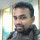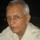Coffee Room
Discuss anything here - everything that you wish to discuss with fellow engineers.
12915 Members
Join this group to post and comment.# Why is capacity inversely proportional to volume?

I would like to know how capacity is inversly proportional to vacuum ? see the graph which is given below.N.SACHIN
I would like to know how capacity is inversly proportional to vacuum ? see the graph which is given below.
What does this graph represent? If it is about a pump, then for a given horse power, the graph is valid. The power consumed by a pump is directly related to the volumetric capacity/time x the vacuum level.Sir it is about vacuum pump.From the above graph i can say that,if capacity increases vacuum decreases i.e how vacuum is inversly proportional to capacity ?I am removing the earlier post as it was a bit confusing. Let me see if this is slightly better. For a vacuum pump the product of the volume of gas pumped in a given time and the pressure difference represents the power. This implies that a high capacity pump will have low pressure difference and vice versa. This the reason for the graph's shape.